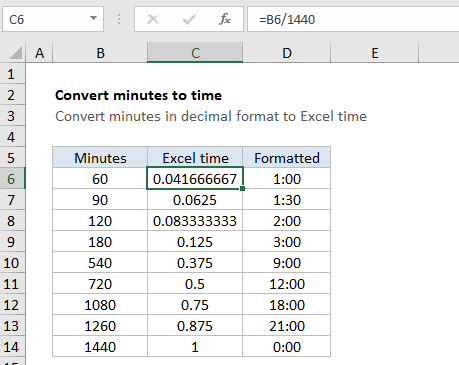## Excel Office

Excel How Tos, Tutorials, Tips & Tricks, Shortcuts

# Convert decimal minutes to Excel time

This tutorial shows how to Convert decimal minutes to Excel time using example below.

To convert minutes in decimal format to a proper Excel time, divide by 1440.

## Formula

`=minutes/1440`## Explanation

In the example shown, the formula in C6 is:

`=B6/1440`

Because B6 contains 60 (representing 360 minutes) the result is 60/1440 = 0.04167, since 60 minutes = 1 hour = 1/24 day. Cell D6 shows the same result formatted as time, which displays 1:00.

### How this formula works

In the Excel date system, one day is equal to 1, so you can think of time as fractional values of 1, as shown in the table below:

Hours Fraction Minutes Value Time
1 1/24 60 0.04167 1:00
3 3/24 180 0.125 3:00
6 6/24 360 0.25 6:00
4 4/24 240 0.167 4:00
8 8/24 480 0.333 8:00
12 12/24 720 0.5 12:00
18 18/24 1080 0.75 18:00
21 21/24 1260 0.875 21:00

This means if you have a decimal number for minutes, you can divide by 1440 (24 x 60) to get the correct representation of minutes in Excel. After dividing by 1440, you can apply a time format of your choice, or use the result in a math operation with other dates or times.

In the example, since B11 contains 720 (representing 720 minutes) the result is 720/1440 = 0.5. Once a time format like h:mm has been applied, Excel will display 12:00.

### Durations

To display hours that represent a duration longer than 24 hours, or minutes in durations longer than 60 minutes, you’ll need to adjust the number format by adding square brackets.

```[h]:mm // hours > 24
[m]  // minutes > 60```

The brackets signal to Excel that the time is a duration, and not a time of day.